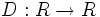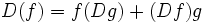# Derivation

Let$R$ be a commutative unital ring (resp. sheaf of commutative unital rings). A derivation of$R$ is a map$D: R \to R$ (resp. a sheaf-theoretic map from$M$ to itself) such that:
•$D$ is$R$-linear (viz, its a map of$R$-modules)
•$D(f) = f(Dg) + (Df)g$